﻿ Flow between plates with different temperatures - XSim

# Flow between plates with different temperatures

Update: June 1, 2017
OpenFOAM 4.x

## Case directory

\$FOAM_TUTORIALS/heatTransfer/buoyantSimpleFoam/buoyantCavity

## Summary

We calculate the flow between two plates with different temperatures. The hot plate is set to 34.6 °C (307.75 K) and the cold plate is set to 15 °C (288.15 K). The gravity acceleration is set to be in the negative direction on the Y-axis.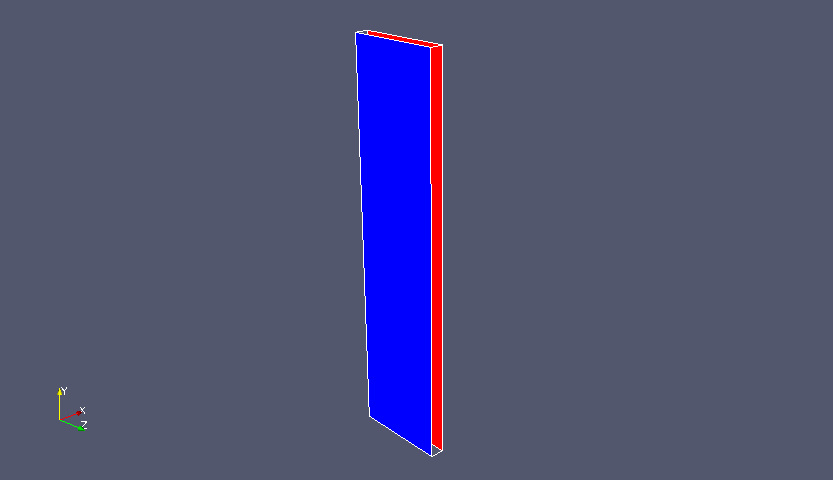Model geometry (Hot side: red, Cold side: blue)

After the calculation, we will sample the values with the utility "postProcess". Sampling settings are specified in the file system/sample.Sampling positions

The magnitude and direction of the gravity acceleration is specified in the file constant/g as follows.

```dimensions      [0 1 -2 0 0 0 0];
value           (0 -9.81 0);
```

The meshes are as follows, and the number of mesh is 78750.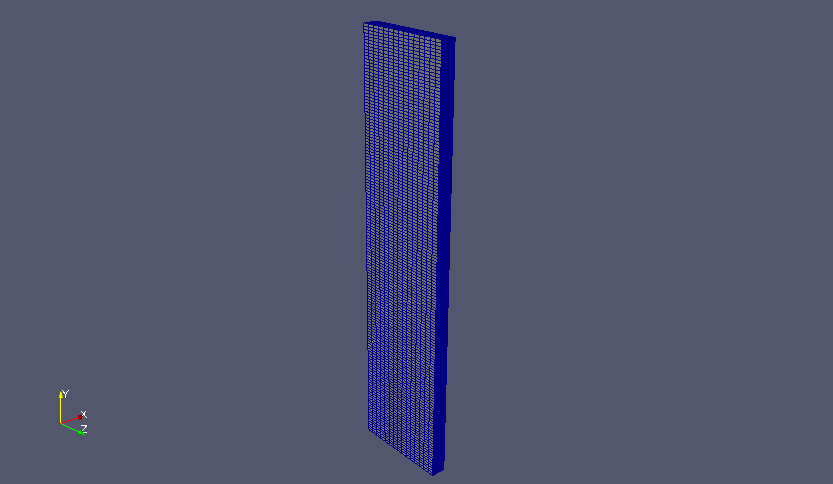Meshes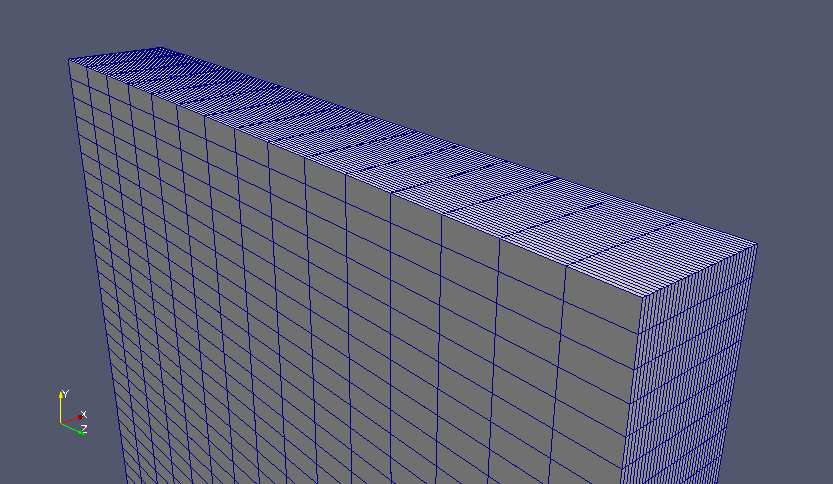Zoomed meshes

The calculation result is as follows.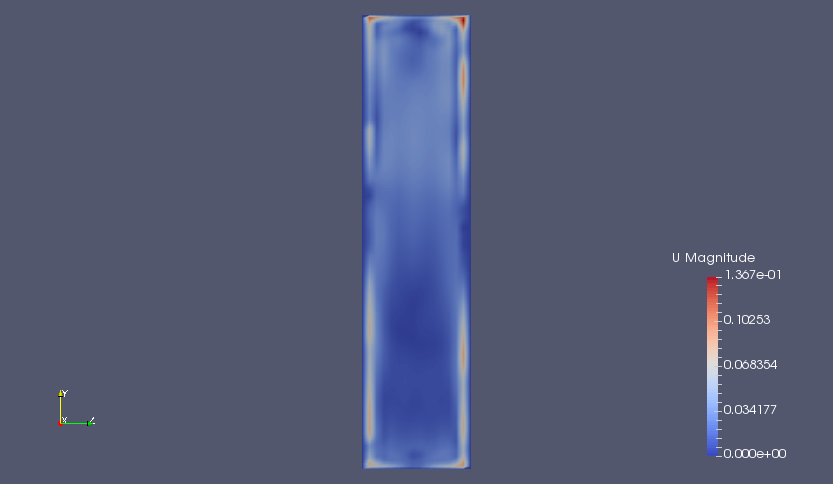Flow velocity on YZ-plane (U)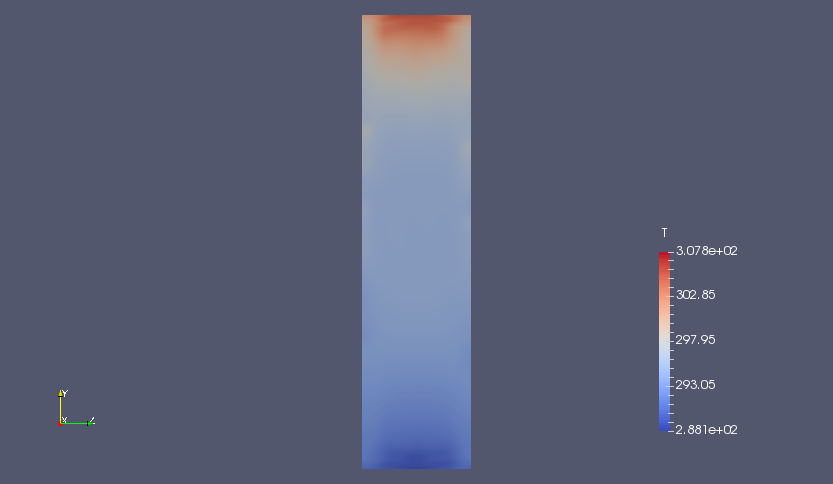Temperature on YZ-plane (T)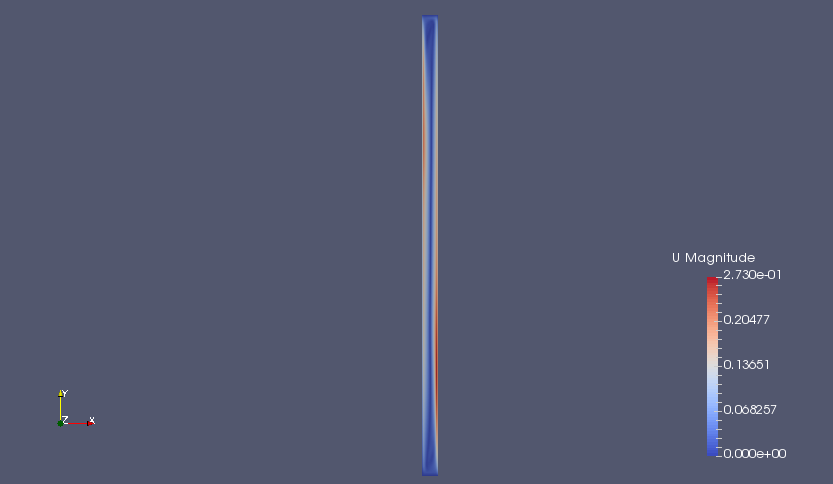Flow velocity on XY-plane (U)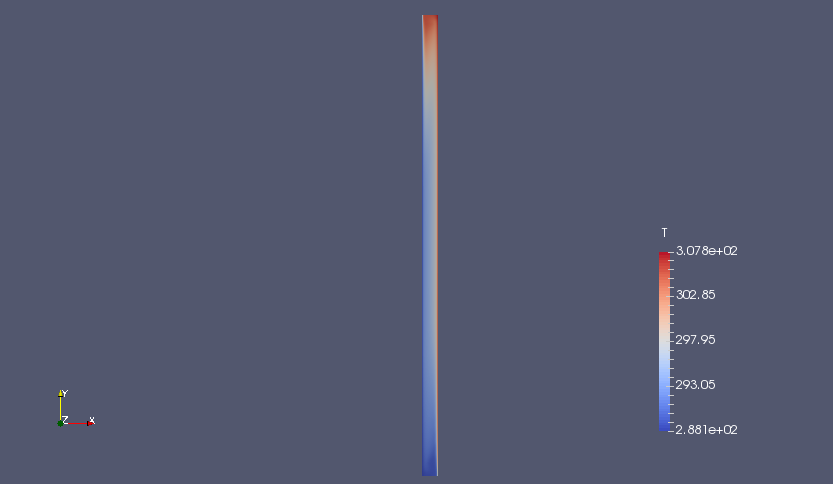Temperature on XY-plane (T)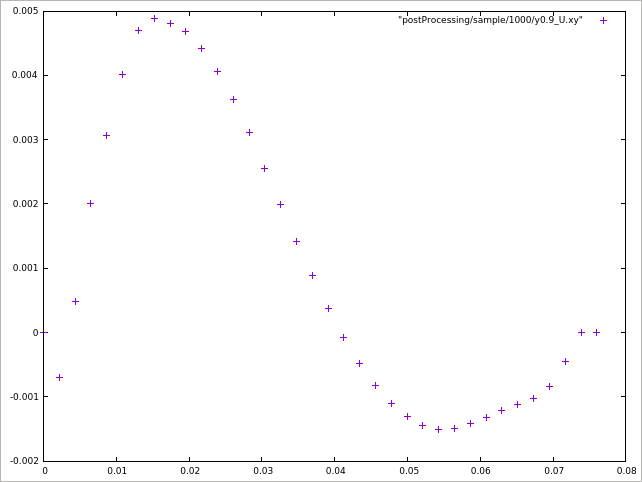Sampled values of flow velocity (U) at y=1.962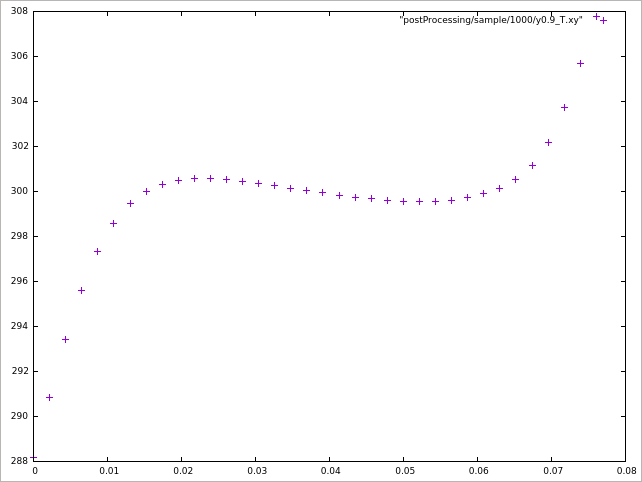Sampled values of temperature (T) at y=1.962

## Commands

cp -r \$FOAM_TUTORIALS/heatTransfer/buoyantSimpleFoam/buoyantCavity buoyantCavity
cd buoyantCavity

blockMesh
buoyantSimpleFoam

postProcess -latestTime -func sample

paraFoam

gnuplot
gnuplot>plot "postProcessing/sample/1000/y0.9_U.xy"

We sampled the values with the utility "postProcess".

## Calculation time

5 minutes 6.86 seconds *Single, Inter(R) Core(TM) i7-2600 CPU @ 3.40GHz 3.40GHz